Next: Stress Tensor in a Up: Mathematical Models of Fluid Previous: Volume and Surface Forces

# General Properties of Stress Tensor

The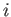-component of the total force acting on a fluid element consisting of a fixed volumeenclosed by a surface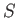is written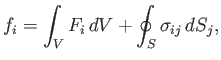(1.4)

where the first term on the right-hand side is the integrated volume force acting throughout, whereas the second term is the net surface force acting across. Making use of the tensor divergence theorem (see Section B.4), the previous expression becomes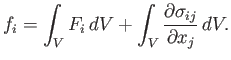(1.5)

In the limit, it is reasonable to suppose that the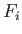and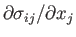are approximately constant across the element. In this situation, both contributions on the right-hand side of the previous equation scale as. According to Newtonian dynamics, the-component of the net force acting on the volume element is equal to the-component of the rate of change of its linear momentum. However, in the limit, the linear acceleration and mass density of the fluid are both approximately constant across the element. In this case, the rate of change of the element's linear momentum also scales as. In other words, the net volume force, surface force, and rate of change of linear momentum of an infinitesimal fluid element all scale as the volume of the element, and consequently remain approximately the same order of magnitude as the volume shrinks to zero. We conclude that the linear equation of motion of an infinitesimal fluid element places no particular restrictions on the stress tensor.

The-component of the total torque, taken about the originof the coordinate system, acting on a fluid element that consists of a fixed volumeenclosed by a surfaceis written [see Equations (A.46) and (B.6)](1.6)

where the first and second terms on the right-hand side are due to volume and surface forces, respectively. [Here,is the third-order permutation tensor. See Equation (B.7).] Making use of the tensor divergence theorem (see Section B.4), the previous expression becomes(1.7)

which reduces to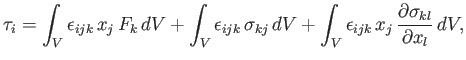(1.8)

because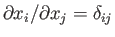. [Here,is the second-order identity tensor. See Equation (B.9).] Assuming that pointlies within the fluid element, and taking the limitin which the,, andare all approximately constant across the element, we deduce that the first, second, and third terms on the right-hand side of the previous equation scale as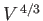,, and, respectively (because). According to Newtonian dynamics, the-component of the total torque acting on the fluid element is equal to the-component of the rate of change of its net angular momentum about. Assuming that the linear acceleration and density of the fluid are approximately constant across the element, we deduce that the rate of change of its angular momentum scales as(because the net rate of change of the linear momentum scales as, so the net rate of change of the angular momentum scales as, and). Hence, it is clear that the rotational equation of motion of a fluid element, surrounding a general point, becomes completely dominated by the second term on the right-hand side of Equation (1.8) in the limit that the volume of the element approaches zero (because this term is a factorlarger than the other terms). It follows that the second term must be identically zero (otherwise an infinitesimal fluid element would acquire an absurdly large angular velocity). This is only possible, for all choices of the position of point, and the shape of the element, if(1.9)

throughout the fluid. The previous relation shows that the stress tensor must be symmetric: that is,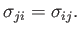(1.10)

It immediately follows that the stress tensor only has six independent components (i.e.,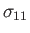,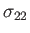,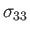,,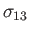, and).

It is always possible to choose the orientation of a set of Cartesian axes in such a manner that the non-diagonal components of a given symmetric second-order tensor field are all set to zero at a given point in space. (See Exercise B.6.) With reference to such principal axes, the diagonal components of the stress tensorbecome so-called principal stresses--,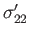,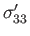, say. In general, the orientation of the principal axes varies with position. The normal stressacting across a surface element perpendicular to the first principal axis corresponds to a tension (or a compression ifis negative) in the direction of that axis. Likewise, forand. Thus, the general state of the fluid, at a particular point in space, can be regarded as a superposition of tensions, or compressions, in three orthogonal directions.

The trace of the stress tensor,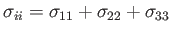, is a scalar, and, therefore, independent of the orientation of the coordinate axes. (See Appendix B.) Thus, it follows that, irrespective of the orientation of the principal axes, the trace of the stress tensor at a given point is always equal to the sum of the principal stresses: that is,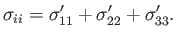(1.11)Next: Stress Tensor in a Up: Mathematical Models of Fluid Previous: Volume and Surface Forces
Richard Fitzpatrick 2016-03-31